# Test Prep Plan - Take a practice test

Take this practice test to check your existing knowledge of the course material. We'll review your answers and create a Test Prep Plan for you based on your results.
How Test Prep Plans work
1
2Based on your results, we'll create a customized Test Prep Plan just for you!
3Study smarter
Study more effectively: skip concepts you already know and focus on what you still need to learn.

# FTCE Math: Trigonometry Chapter Exam

Exam Instructions:

Choose your answers to the questions and click 'Next' to see the next set of questions. You can skip questions if you would like and come back to them later with the yellow "Go To First Skipped Question" button. When you have completed the practice exam, a green submit button will appear. Click it to see your results. Good luck!

### Page 1

#### Question 1 1. Solve for the value of x in the trigonometric equation below. (Use the domain -π/2 to π/2)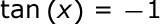#### Question 2 2. In the pictured triangle, side b is 12 and side c is 16. If ∠A is 84 degrees, then approximately how long is side a? Round the value of the cosine to the nearest whole number.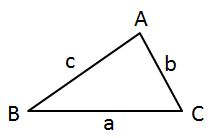#### Question 4 4. In the pictured triangle, ∠A is 62 degrees and ∠C is 86 degrees. If side a is 112 units long, approximately how long is side c?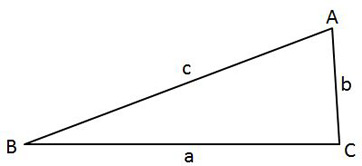#### Question 5 5. In the pictured triangle, ∠A is 58 degrees and ∠C is 63 degrees. If side a is 84 units long, approximately how long is side c?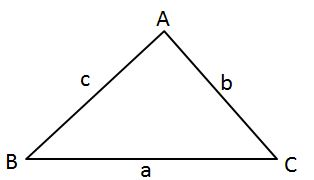### Page 2

#### Question 6 6. In the pictured triangle, side a is 17 and side c is 20. If ∠B is 19 degrees, then approximately how long is side b? Round the value of the cosine to the nearest whole number.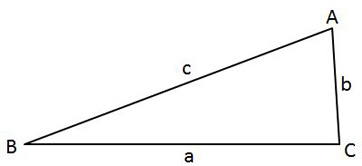#### Question 8 8. In the pictured triangle, ∠A is 137 degrees and ∠B is 28 degrees. If side b is 71 units long, approximately how long is side a?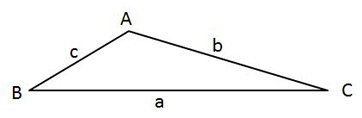#### Question 9 9. Solve the following equation for x.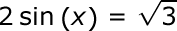#### Question 10 10. In the pictured triangle, ∠A is 98 degrees and ∠B is 12 degrees. If side a is 84 units long, approximately how long is side b?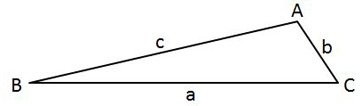### Page 3

#### Question 12 12. In the pictured triangle, what ratio represents the cosine of theta?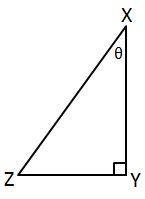#### Question 13 13. Simplify the following expression.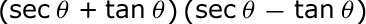### Page 4

#### Question 16 16. What is x?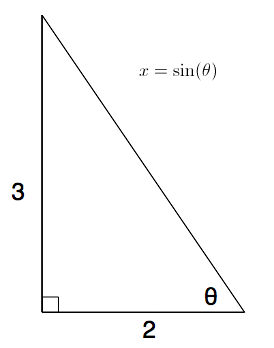#### Question 17 17. Solve the following equation for θ.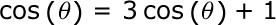#### Question 18 18. What's the value of sec2 (θ) given that the equation below is true?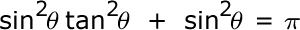#### Question 19 19. What is z in terms of x?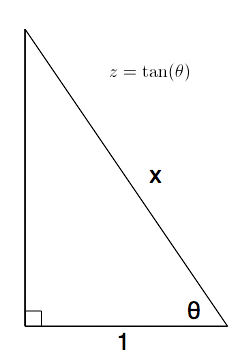### Page 5

#### Question 24 24. Solve the following trigonometric equation for x.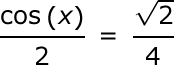#### Question 25 25. Solve the following trigonometric equation for θ.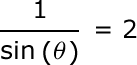### Page 6

#### Question 29 29. What is x?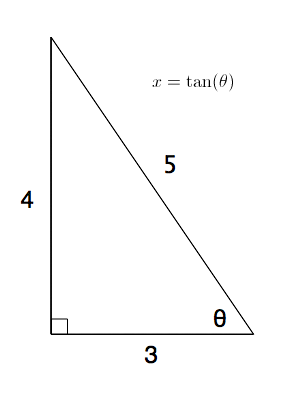#### Question 30 30. What is the value of cos(θ) given that the equation below is true?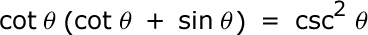#### FTCE Math: Trigonometry Chapter Exam Instructions

Choose your answers to the questions and click 'Next' to see the next set of questions. You can skip questions if you would like and come back to them later with the yellow "Go To First Skipped Question" button. When you have completed the practice exam, a green submit button will appear. Click it to see your results. Good luck!

Support